﻿ What is Effective or APR rate
WinAmort Professional 2.0

Effective Rate or Annual Percentage Rate (APR)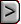Effective Rate or Annual Percentage Rate (APR)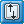Effective Rate or Annual Percentage Rate (APR)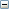What is the APR - Annual Percentage Rate?
 The Annual Percentage Rate is the true interest rate being charged when a lender charges the borrower an extra penalty in the form of a one-time up front cost, or a fee every time a payment is made towards the borrowed funds. The Annual Percentage Rate window will appear if you press the button found on the right side of the screen. The Annual Percentage Rate is a result of the Truth in Lending Act, in America. Some lending institutions charge their customers points on loans taken out. Points are percentage points charged to principal amounts when a lender wants to value risk loans. For example if 2 points are charged on a \$100,000 dollar loan, this equals \$2,000 fee. Therefore, when the lender gives a borrower 2 points on the loan, is translates into charging the borrower \$2,000 in penalty for borrowing the funds. Now this penalty is usually taken out of the principal amount being borrowed. So when the borrower wanted to borrow \$100,000 and was charged 2 points on the loan, he in essence only received \$98,000. The other \$2,000 is a fee already collected by the lender. Now if the lender agreed to loan out the principal amount for 10%, this rate was based on the entire \$100,000. But because the borrower is being charged \$2,000 fee up front he is only receiving \$98,000 yet has to pay back \$100,000 and is charged interest on this amount.   Although it may sound confusing, what is really occurring is that interest is being quoted on \$100,000 and only \$98,000 is being received. Therefore, the interest rate being charged in not correctly being represented. In order to force the lender to display the correct amount of the interest rate, the American Government passed an act called the Truth in Lending Act. This forces the lender to show the correct interest rate that is being charged. Less principal is being given to the borrower, yet the interest rate is based on a higher principal amount, a calculation is needed to determine what is called the Annual Percentage Rate or in other words the actual interest rate. Simply Interest Professional will easily determine the correct APR rate as needed in order to meet the Truth in Lending Act.   The situation of points described is just one of the factors that results in a technically in correct interest rate value. Other factors are fees with each payment. Each point is easily accommodated by Simply Interest Professional as we are about to see in the following example.   Each point under a point system, represents 1% of the face amount of the mortgage or loan note. In a typical point-system loan, the lender assesses a specified number of points to each note. This reduces the amount of cash the borrower receives to some amount below the face amount of the note. Thus, each point assessed increases the actual interest rate to some amount above that stated for the note. This Annual Percentage Rate. must be reported by all lending institutions as stated by Regulation Z - Truth in Lending Act in the United States.

Example question for to compute APR.

 APR - Example XYZ Company takes out a 15 year, \$147,000 loan on August 1, 2011 at an annual interest rate of 10.75%. The lending institution charges 2 points on the loan. The first payment is due on September 1, 2011.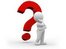Solve The Payment amount first.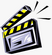Enter the data as shown in the screen below: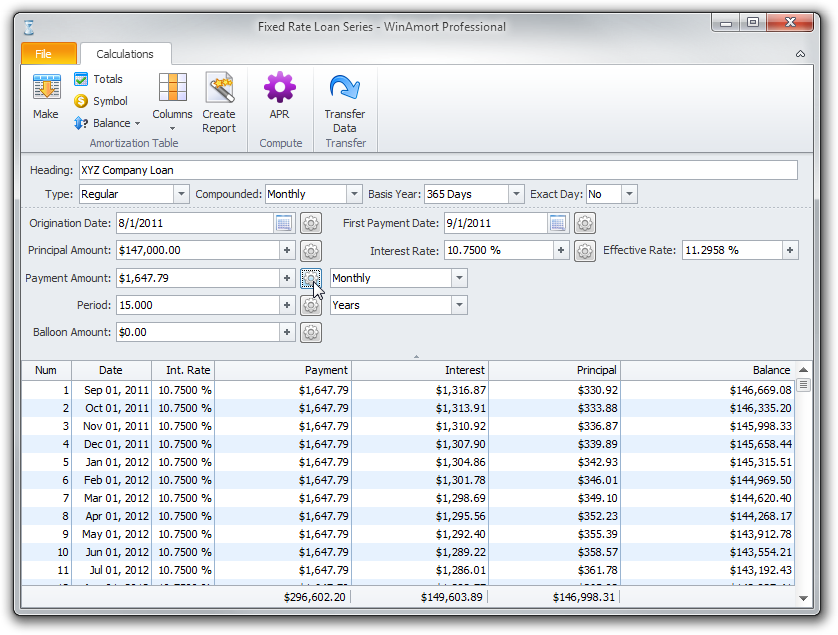Press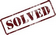Press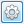button beside the Payment field to compute the answer.     The monthly payment is \$1647.79.     Now we will compute the APR.     APR button at the top of the form.

The following new window will appear.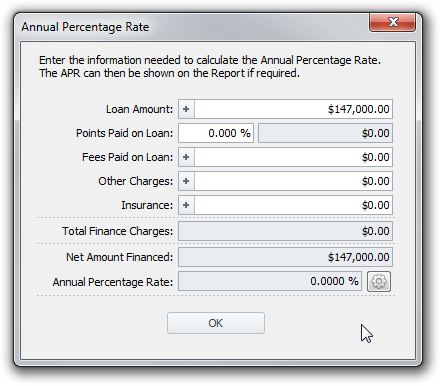Type                               Press2.000 in the Points and Fees field as shown above.   The Points amount of \$2940.00 will instantly be computed and subtracted from the \$147,000 Principal amount to give the actual amount financed.   The Fees Paid on Loan field can be used for the loans that do not state the fees in points, but instead give the fee amount in dollars. Entering the fees in points, or its equivalent in dollars makes no difference in the computation of the APR.   The Fee with each Payment field can be used to compute the APR for  loans for which the lending institution charges a fee with each payment. Just enter the fee amount in the Fee with each Payment field and press the COMPUTE button. The fee with each payment is a finance charge that effectively reduces the amount actually borrowed and thus causes the Annual Percentage Rate to increase. You do not need it for this example.button beside the Annual Percentage Rate field to compute the answer.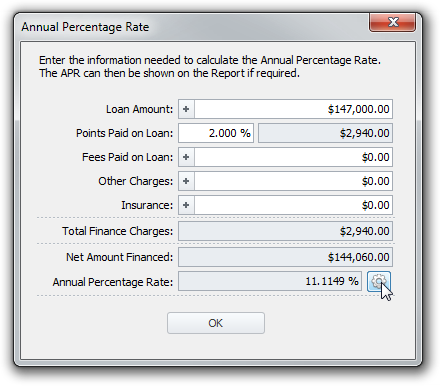The Annual Percentage Rate is 11.1149%